# Continuous Charge Distribution

We have dealt with the systems containing discrete charges such as Q1, Q2,…, Qn. We noticed that the operations involved in such cases were simpler and rules of scalar addition could be easily applied. But as we know, at times we need to deal with systems where considering the charges discrete is not an option. Here, we need to consider the charge as those which are continuously distributed over a length or a surface or a volume. For example, if we are dealing with a surface carrying a continuous charge distribution in the body over its surface, we cannot calculate the value of the electric field due to each microscopic charged constituent. We rather consider an area element, very small, but big enough to include many such charged constituents which we can denote as Δs.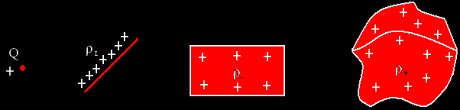Let us say the total charge carried by this area element is equal to ΔQ, and then the charge density of the element can be given as,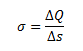The unit of σ is C/m2 or Coulomb per square metres.

Similarly, in the case of charge distribution in a body along a line segment of length Δl such as a small line element of wire, the linear charge density can be given as,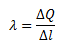Where ΔQ is the charge contained in that line element. The unit of λ is C/m or Coulomb per meter.

Similarly, when the charge ΔQ is distributed over a microscopically small volume element ΔV, the volume charge density can be given as,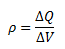The unit of ρ is C/m3or Coulomb per cubic metre.

## Calculation of Electric Field

Let us consider a case of the continuous charge distribution in a body. Here, we will calculate the electric field due to this charge at a point P. We can say that the charge density at different volumetric elements can be different, so we divide the body into different elements such that the charge density for a particular element can be considered to be a fixed quantity. Consider one such element of volume Δv, whose charge density is given by ρ. Let the distance of the volume element from the point P be given as r. The charge in the volume element can be given as ρΔv. As per the Coulomb’s law, the electric field due to the charge ρΔv can be given as,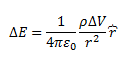Here, r is the distance between the charged element and the point P at which the field is to be calculated and ř is the unit vector in the direction of the electric field from the charge to the point P.

By the principle of superposition, the electric field due to the total charge distribution in a body, distributed into several such volume elements, can be given as,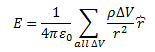## Frequently Asked Questions – FAQs

Q1

### How are electric charges distributed within the atom?

Subatomic particles carry electric charges. Electrons carry the negative charge and protons carry the positive charge in the nuclei of atoms.
Q2

### What is charge density?

The charge density is the measure of electric charge per unit area of a surface, or per unit volume of a body or field. It tells us how much charge is stored in a particular field. Charge density can be determined in terms of volume, area, or length.
Q3

### What is continuous charge distribution?

In a continuous charge distribution, all the charges are closely bound together i.e. having very less space between them. But this closely bound system doesn’t mean that the electric charge is uninterrupted. It clears that the distribution of separate charges is continuous, having a minor space between them.
Q4

### What are the types of continuous charged distributions?

Types of continuous charged distributions:
1. Linear Charge Distribution
2. Surface Charge Distribution
3. Volume Distribution of charge
Q5

### What is symmetrical charge distribution?

A symmetrical charge distribution is when there is a couple of geometrical transformations such that these transformations do not affect any physical change.

Stay tuned with BYJU’S for more such interesting articles. Also, register to “BYJU’S – The Learning App” for loads of interactive, engaging Physics-related videos and an unlimited academic assist.

Test Your Knowledge On Continuous Charge Distribution!